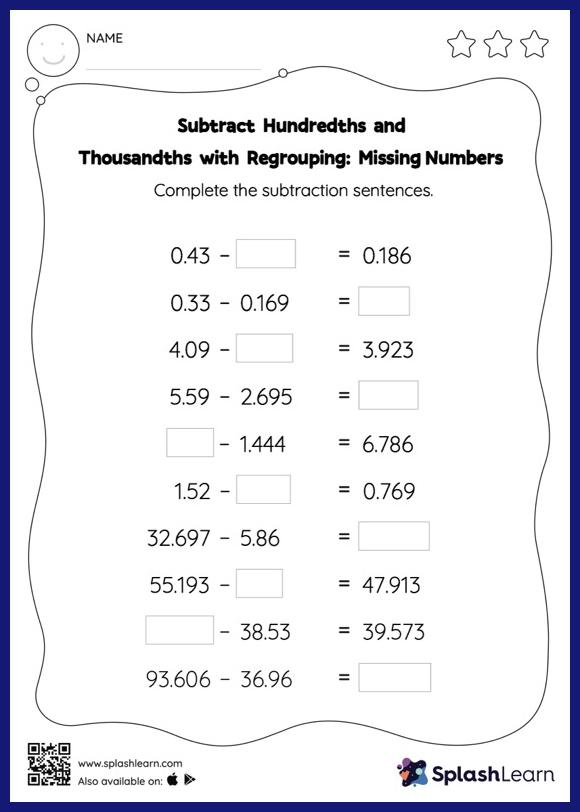# Subtract Hundredths and Thousandths with Regrouping: Missing Numbers Worksheet

Home > Subtract Hundredths and Thousandths with Regrouping: Missing NumbersThis subtract hundredths and thousandths with regrouping worksheet consists of a set of questions on subtraction, which help students develop fluency. Students find the missing number while subtracting decimals by using the relationship between addition and subtraction. To reach the answer, they also use the relationship between ones, tenths, hundredths, thousandths, etc., To regroup the numbers in subtract hundredths and thousandths with regrouping worksheet. In each problem, the numbers are laid out in the horizontal format. Students should try to use different strategies involving composing and decomposing numbers to solve these problems. This will help them develop flexibility and fluency.